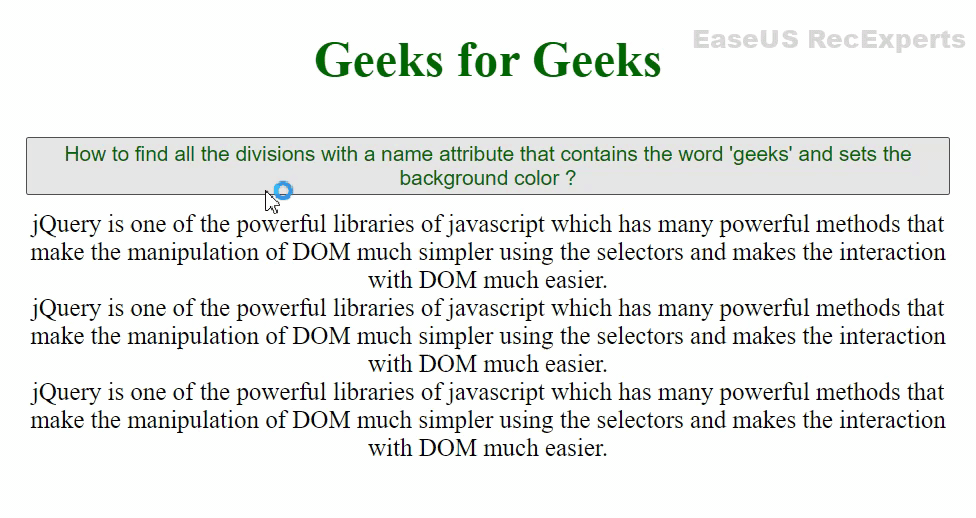Related Articles

# How to find all divs with a name attribute that contains word ‘geeks’ and sets the background color ?

• Last Updated : 02 Jul, 2021

jQuery contains attribute selectors with the help of which we can select the elements with name attribute as a particular string or select the elements which contain a particular string or begin with a particular string or ends with a particular string or doesn’t contain particular string as a name attribute.

In this article, we will learn to find all the divisions with a name attribute that contains the word ‘geeks’ and sets the background colour green using jQuery attribute selectors.

Approach1: To find the name attribute that contains a particular string, we can use an attribute selector with ~ to select all the elements that contain a word with name attribute as geeks.

Syntax:

`\$("[attribute~='word']");`

HTML Code:

## HTML

 ```<``html` `lang``=``"en"``>`` ` `<``head``>``    ``<``meta` `charset``=``"UTF-8"``>``    ``<``meta` `http-equiv``=``"X-UA-Compatible"` `content``=``"IE=edge"``>``    ``<``meta` `name``=``"viewport"` `          ``content``=``"width=device-width, initial-scale=1.0"``>``    ` `    ````    ``<``script` `src``=``"https://code.jquery.com/jquery-3.6.0.min.js"``            ``integrity``=``    ``"sha256-/xUj+3OJU5yExlq6GSYGSHk7tPXikynS7ogEvDej/m4="` `            ``crossorigin``=``"anonymous"``>``    ````    ``<``style``>``        ``h1 {``            ``color: #006600;``            ``text-align: center;``        ``}``        ` `        ``.bg-green {``            ``background-color: #006600;``            ``color: white; ``            ``margin: 2px;``            ``border: 2px solid black; ``        ``}``        ``#btn {``            ``color: #006600;``            ``text-align: center;``            ``margin: 10px;``        ``}``        ``body {``            ``text-align : center;``        ``}``    `````` ` `<``body``>``    ``<``h1``> Geeks for Geeks``    ``<``button` `id``= ``"btn"``>``        ``How to find all the divisions with a name ``        ``attribute that contains the word 'geeks'``        ``and sets the background color?``    ```` ` `    ``<``div` `name` `= ``"geeks for geeks"``>``        ``jQuery is one of the powerful libraries ``        ``of javascript which has many powerful ``        ``methods that make the manipulation of ``        ``DOM much simpler using the selectors and``        ``makes the interaction with DOM much easier.``    ```` ` `    ``<``div` `name` `= ``"gfg"``>``        ``jQuery is one of the powerful libraries of ``        ``javascript which has many powerful methods ``        ``that make the manipulation of DOM much simpler``        ``using the selectors and makes the interaction ``        ``with DOM much easier.``    ```` ` `    ``<``div` `name` `= ``"geeks"``>``        ``jQuery is one of the powerful libraries of ``        ``javascript which has many powerful methods ``        ``that make the manipulation of DOM much simpler``        ``using the selectors and makes the interaction ``        ``with DOM much easier.``  ```` ` `    ``<``script``>``        ``\$(document).ready(function () {``            ``\$('#btn').click(function(){``           ``\$("div[name~='geeks']").addClass('bg-green');``            ``});``        ``});    ``    `````` ` ``

Output:Approach 2: Using * for selecting all divs that contain word “geeks” but the difference between the ~ and * is that ~ selects words separated by spaces. For * there need not be any spaces, it can select even a substring.

Syntax:

`\$("[attribute*='word']");`

HTML Code:

## HTML

 ```<``html` `lang``=``"en"``>`` ` `<``head``>``    ``<``meta` `charset``=``"UTF-8"``>``    ``<``meta` `http-equiv``=``"X-UA-Compatible"` `content``=``"IE=edge"``>``    ``<``meta` `name``=``"viewport"` `          ``content``=``"width=device-width, initial-scale=1.0"``>``    ` `    ````    ``<``script` `src``=``"https://code.jquery.com/jquery-3.6.0.min.js"``        ``integrity``=``    ``"sha256-/xUj+3OJU5yExlq6GSYGSHk7tPXikynS7ogEvDej/m4="` `        ``crossorigin``=``"anonymous"``>``    ```` ` `    ``<``style``>``        ``h1 {``            ``color: #006600;``            ``text-align: center;``        ``}``        ` `        ``.bg-green {``            ``background-color: #006600;``            ``color: white; ``            ``margin: 2px;``            ``border: 2px solid black; ``        ``}``        ``#btn {``            ``color: #006600;``            ``text-align: center;``            ``margin: 10px;``        ``}``        ``body {``            ``text-align : center;``        ``}``    `````` ` `<``body``>``    ``<``h1``> Geeks for Geeks``    ``<``button` `id``= ``"btn"``> ``       ``How to find all the divisions with a name ``       ``attribute that contains the word 'geeks'``       ``and sets the background color?``   ```` ` `    ``<``div` `name` `= ``"geeks for geeks"``>``        ``jQuery is one of the powerful libraries of ``        ``javascript which has many powerful methods ``        ``that make the manipulation of DOM much simpler``        ``using the selectors and makes the interaction ``        ``with DOM much easier.``    ```` ` `    ``<``div` `name` `= ``"gfg"``>``        ``jQuery is one of the powerful libraries of ``        ``javascript which has many powerful methods ``        ``that make the manipulation of DOM much simpler``        ``using the selectors and makes the interaction ``        ``with DOM much easier.``    ```` ` `    ``<``div` `name` `= ``"geeks"``>``        ``jQuery is one of the powerful libraries of ``        ``javascript which has many powerful methods ``        ``that make the manipulation of DOM much simpler``        ``using the selectors and makes the interaction ``        ``with DOM much easier.``    ```` ` `    ``<``script``>``        ``\$(document).ready(function () {``            ``\$('#btn').click(function(){``           ``\$("div[name*='geeks']").addClass('bg-green');``            ``});``        ``});    ``    `````` ` ``

Output:Attention reader! Don’t stop learning now. Get hold of all the important HTML concepts with the Web Design for Beginners | HTML  course.

My Personal Notes arrow_drop_up